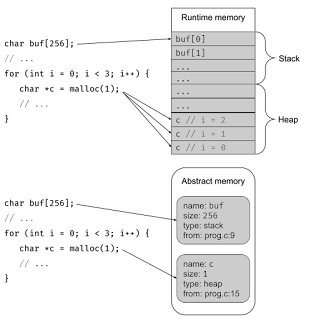## What are Virtual Calls in C++?

Virtual functions are an essential feature of object-oriented programming (OOP) in C++. Virtual functions enable polymorphism, which allows us to write code that can work with objects of different types, as long as they are derived from the same base class.

A virtual function is a function that is declared in the base class with the virtual keyword and is overridden by a derived class. When a virtual function is called on a pointer or reference to an object, the function that is called depends on the type of the object pointed or referenced, not the type of the pointer or reference itself.

## Example 1: Virtual Function in C++

In this example, we will create a base class called "Shape" and two derived classes called "Circle" and "Square". Each class will have a virtual function called "area()" that will calculate the area of the respective shape.

`C++`
``````#include <iostream>

using namespace std;

class Shape {
public:
virtual float area() {
cout << "Parent class area." << endl;
return 0;
}
};

class Circle : public Shape {
public:

Circle(float r) {
}

float area() {
}
};

class Square : public Shape {
public:
float side;

Square(float s) {
side = s;
}

float area() {
return side * side;
}
};

int main() {
Shape *shape;
Circle c(5);
Square s(10);

shape = &c;
cout << "Area of Circle: " << shape->area() << endl;

shape = &s;
cout << "Area of Square: " << shape->area() << endl;

return 0;
}
``````

In this example, we define a base class "Shape" with a virtual function "area()". We also define two derived classes "Circle" and "Square" that inherit from the "Shape" class and override the "area()" function to calculate the area of a circle and square, respectively.

In the main function, we create objects of the "Circle" and "Square" classes and assign their addresses to a pointer of the base class "Shape". When we call the "area()" function through the base class pointer, the appropriate function is called based on the actual type of the object pointed to.

## Example 2: Virtual Destructor in C++

In this example, we will create a base class called "Base" and a derived class called "Derived". Both classes will have a virtual destructor.

`C++`
``````#include <iostream>

using namespace std;

class Base {
public:
Base() {
cout << "Constructing Base." << endl;
}

virtual ~Base() {
cout << "Destructing Base." << endl;
}
};

class Derived : public Base {
public:
Derived() {
cout << "Constructing Derived." << endl;
}

~Derived() {
cout << "Destructing Derived." << endl;
}
};

int main() {
Base *b = new Derived();
delete b;

return 0;
}``````

In this example, we define a base class "Base" with a virtual destructor and a derived class "Derived" that also has a virtual destructor. In the main function, we create a pointer to the base class "Base" that points to an object of the derived class "Derived". When we deletethe object using the pointer to the base class, the derived class destructor is called first and then the base class destructor is called. This is necessary to ensure that the memory allocated to the derived class is properly deallocated.

## Example 3: Pure Virtual Function in C++

In this example, we will create an abstract base class called "Shape" and two derived classes called "Circle" and "Square". The "Shape" class will have a pure virtual function called "area()" that will be overridden by the derived classes.

`C++`
``````#include <iostream>

using namespace std;

class Shape {
public:
virtual float area() = 0;
};

class Circle : public Shape {
public:

Circle(float r) {
}

float area() {
}
};

class Square : public Shape {
public:
float side;

Square(float s) {
side = s;
}

float area() {
return side * side;
}
};

int main() {
Shape *shape;
Circle c(5);
Square s(10);

shape = &c;
cout << "Area of Circle: " << shape->area() << endl;

shape = &s;
cout << "Area of Square: " << shape->area() << endl;

return 0;
}
``````

In this example, we define an abstract base class "Shape" with a pure virtual function "area()". A pure virtual function is a virtual function that has no implementation in the base class and must be overridden by the derived classes. We also define two derived classes "Circle" and "Square" that inherit from the "Shape" class and override the "area()" function to calculate the area of a circle and square, respectively.

In the main function, we create objects of the "Circle" and "Square" classes and assign their addresses to a pointer of the base class "Shape". When we call the "area()" function through the base class pointer, the appropriate function is called based on the actual type of the object pointed to.

## Conclusion

In conclusion, virtual functions and virtual calls are an essential feature of object-oriented programming in C++. They allow us to write code that can work with objects of different types as long as they are derived from the same base class. In this post, we provided a step-by-step guide along with three examples that beginners can follow to understand virtual calls in C++. We hope this post has been helpful to you!